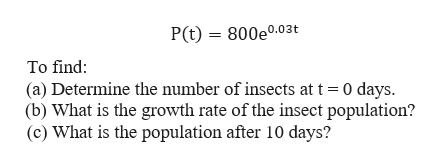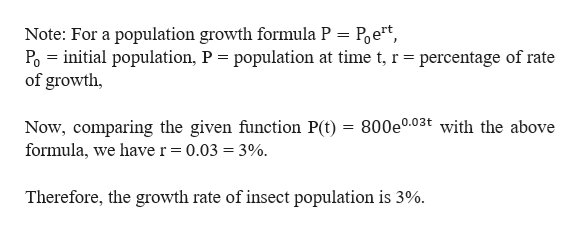# The size P of a certain insect population at time t​ (in days) obeys the function Upper P left parenthesis t right parenthesis equals 800 e Superscript 0.03 t Baseline .P(t)=800e0.03t.​(a) Determine the number of insects att=0 days.​(b) What is the growth rate of the insect​ population?​(c) What is the population after 10​ days?

Question
75 views
The size P of a certain insect population at time t​ (in days) obeys the function Upper P left parenthesis t right parenthesis equals 800 e Superscript 0.03 t Baseline .P(t)=800e0.03t.
​(a) Determine the number of insects at
t=0 days.
​(b) What is the growth rate of the insect​ population?
​(c) What is the population after 10​ days?
check_circle

Step 1

Given that the size P of a certain insect population at time t​ (in days) obeys the function as below:help_outlineImage TranscriptioncloseP(t) 800e0.03t To find: (a) Determine the number of insects at t 0 days. (b) What is the growth rate of the insect population? (c) What is the population after 10 days? fullscreen
Step 2

a)To find number of insects at t = 0 days, we substitute t as 0 in the given function P(t).

Step 3

b) To determine the growth rate of the insect popu...help_outlineImage TranscriptioncloseNote: For a population growth formula P = Poet Po initial population, P = population at time t, r = percentage of rate of growth, Now, comparing the given function P(t) = 800e0.03t with the above formula, we have r = 0.03 = 3%. Therefore, the growth rate of insect population is 3%. fullscreen

### Want to see the full answer?

See Solution

#### Want to see this answer and more?

Solutions are written by subject experts who are available 24/7. Questions are typically answered within 1 hour.*

See Solution
*Response times may vary by subject and question.
Tagged in

### Exponents